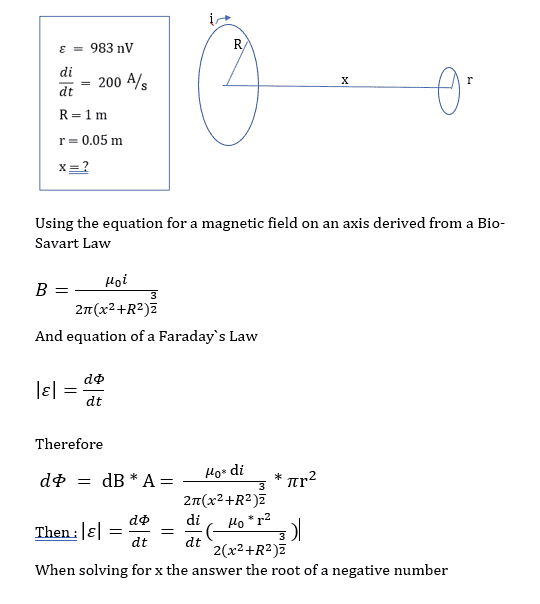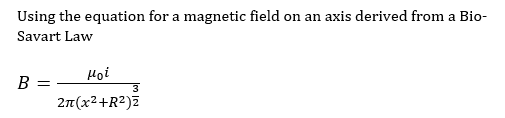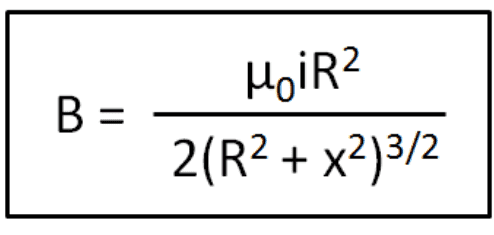# Faraday's law and Magnetic Fields

• maksym_slnc

#### maksym_slnc

Homework Statement
A very large loop of metal wire with radius 1 meter is driven with a linearly increasing current at a rate of 200 amps/second . A very small metal wire loop with radius 5 cm is positioned a small distance away with its center on the same axis (the loops are coaxial). The small loop experiences an induced emf of 983nV . What is the separation of the loops in meters?
Relevant Equations
$$\varepsilon=\oint \overrightarrow{\mathbf{E}} \cdot d \overrightarrow{\mathbf{l}}=-\frac{d \Phi_{\mathrm{m}}}{d t} .$$
${\mathbf{B}}=\frac{\mu_0 I \hat{\mathbf{j}}}{2 \pi\left(y^2+R^2\right)^{3 / 2}}$I am not really sure which direction i am moving in with this solutionCheck this formula. Note that that the right-hand side does not have the correct dimensions for magnetic field. Also, check the numerical factors in the equation. For ##x = 0## the formula should reduce to the field at the center of a circular current loop.